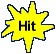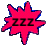Compound Interest - Accumulated Amount - Car Race Theme - Game Tips:

- The compound amount A , can be calculated using the formula A = P(1+i)n
where P is the principal amount invested (Example: \$200.)
i is the interest rate per conversion period (Example: 4% = .04)
n is the number of conversion periods (Example: 5 years).

- Example 1: The compound amount that results from investing \$200 for 5 years
at the interest rate of 4% compounded annually is calculated as A = 200(1+.04)5.
The expression A = 200(1.04)5 can be evaluated using a calculator to get A = 243.33 dollars.
The calculator should have a button to do exponents. (Sample buttons: xy , ax , yx etc.)

- Example 2: The compound amount that results from investing \$200 for 5 years
at the interest rate of 4% compounded quarterly is calculated as A = 200(1+.01)20.
The expression A = 200(1.01)20 can be evaluated using a calculator to get A = 244.04 dollars.
Interest rates (such as 4%) are quoted per annum unless otherwise stated.

- Your Game Score is reduced by the number of car hits.

- To slow the game speed repeat tap/click on the word Slider.
- To increase the game speed repeat tap/click on the word Math.
- Speed can also be adjusted with a keyboard's - and + keys.

- Refresh/Reload the web page to restart the game.